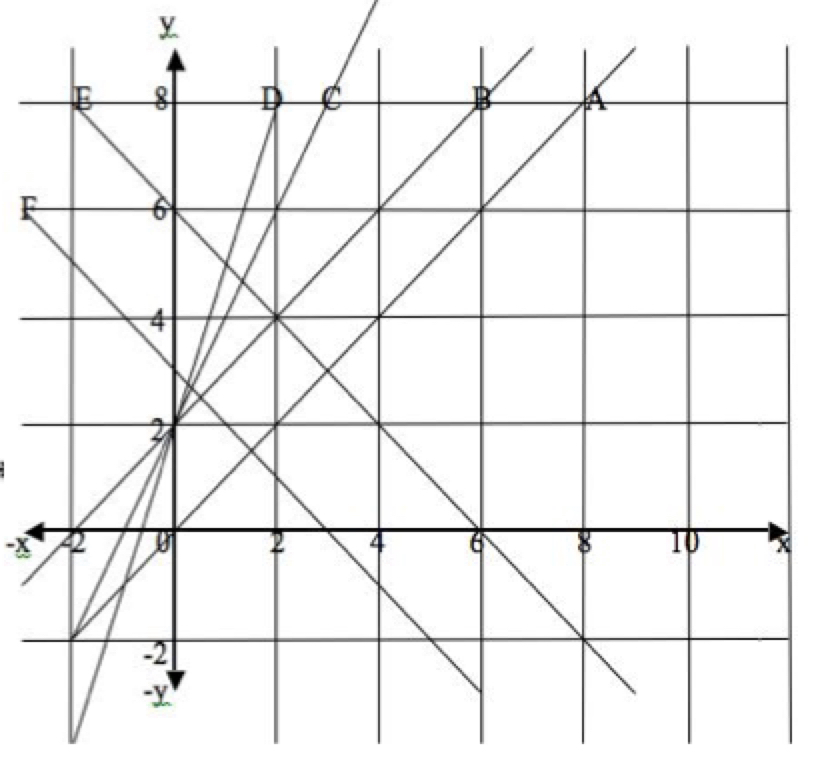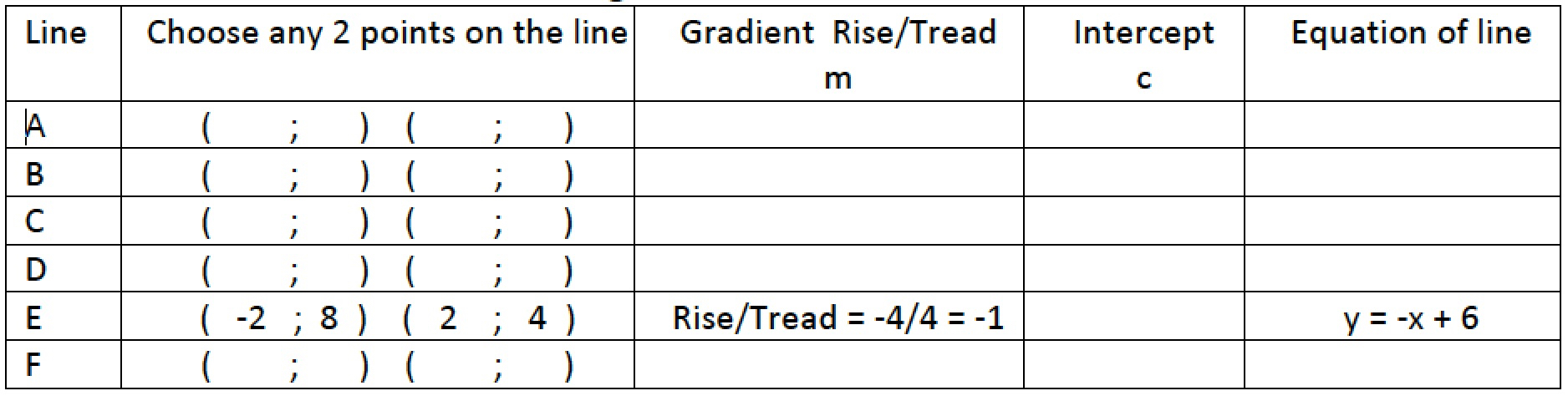1. For each line fill in your answers to the following questions in the table below: (Line E has been done for you.)

(i) Write down the coordinates (0; c) of the point of intersection of the line with the y-axis (the y intercept).

(ii) Choose two points on the line $$(x_1;y_1), (x_2;y_2)$$. Find the gradient of the line from the formula $$m=\frac{y_2-y_1)}{x_2-x_1)}$$.

(iii) Use the values of c and m you have found to write the equation of the line in the form $$y = mx + c$$.

2. What do you notice when you compare the equations of these lines?3. Copy the graphs below and fill in the coordinates of the points.

What are the gradients of the x-axis, the y-axis and the other 4 lines shown?

Write down the equations of the x-axis, the y-axis and the other 4 lines shown.

Tagged with:

### One Response to Grades 10 and 11 Lines

1.Nontsikelelo says:

Thanks a lot for seeing this site. I will visit it frequently.